nextnano.com  GUI: nextnanomat  Tool: nextnano++  Tool: nextnano³  Tool: nextnano.NEGF  Download | Search | Copyright | News | Publications  * password protected nextnano³ software3D cubic QD

# nextnano3 - Tutorial

## Energy levels in idealistic 3D cubic and cuboidal shaped quantum dots

Author: Stefan Birner

If you want to obtain the input files that are used within this tutorial, please contact stefan.birner@nextnano.de.
```-> 3DcubicQD.in  - ```10 nm x 10 nm x 10 nm QD
```-> 3DcuboidQD.in - ```10 nm x 10 nm x   5 nm QD

## Energy levels in an idealistic 3D cubic quantum dot

```-> 3DcubicQD.in  - ```10 nm x 10 nm x 10 nm QD

Here, we want to calculate the energy levels and the wave functions of a cubic quantum dot of length 10 nm.

We assume that the barriers at the QD boundaries are infinite. This way we can compare our numerical calculations to analytical results.
The potential inside the QD is assumed to be 0 eV.

As effective mass we take the electron effective mass of InAs, i.e. me = 0.026 m0.
` conduction-band-masses = 0.026d0  0.026d0  0.026d0  ! `electron effective mass at Gamma conduction band```                           ...```

A discussion of the analytical solution of the 3D Schrödinger equation of a particle in a box (i.e. quantum dot) with infinite barriers can be found in e.g.
Quantum Heterostructures (Microelectronics and Optoelectronics) by V.V. Mitin, V.A. Kochelap and M.A. Stroscio.

The solution of the Schrödinger equation leads to the following eigenvalues:

En1,n2,n3 = hbar2 pi2 / 2me               ( n12 / Lx2 + n22 / Ly2n32 / Lz2 ) =
= 1.4462697 * 10-17 eVm2 ( n12 / Lx2 + n22 / Ly2n32 / Lz2 ) =
= 0.1446269  eV               ( n12           + n22        +  n32         )           (if Lx = Ly = Lz = 10 nm)

• En1,n2,n3 is the total electron energy.
• n1, n2, n3 are three discrete quantum numbers (because we have three directions of quantization).
• Lx, Ly, Lz are the lengths along the x, y and z directions. In our case, Lx = Ly = Lz = 10 nm.

Generally, the energy levels are not degenerate, i.e. all energies are different.
However, some energy levels with different quantum numbers coincide, if the lengths along two or three directions are identical or
if their ratios are integers. In our cubic QD case, all three lengths are identical.
Consequently, we expect the following degeneracies:

• E111 = 0.43388 eV (ground state)
• E112 = E121 = E211 = 0.86776 eV = 2 E111
• E122 = E212 = E221 = 1.30164 eV = 3 E111
• E113 = E131 = E311 = 1.59090 eV = 11/3 E111
• E222 = 1.73552 eV = 4 E111
• E123 = E132 = E213 = E231 = E312 = E321 = 2.02478 eV = 14/3 E111
• E333 = 3.90493 eV = 27/3 E111

nextnano³ numerical results for a 10 nm cubic quantum dot with 0.50 nm grid spacing:
(The grid spacing is rather coarse but has the advantage that the calculation takes only a minute.)

Output file name:``` Schroedinger_1band/ev3D_cb001_qc001_sg001_deg001_dir_Kx001_Ky001_Kz001.dat```

``` num_ev: eigenvalue [eV]:         (0.50 nm grid)   1      0.432989 ```= E111```   2      0.862425      ```(three-fold degenerate)` `E112/E121/E211```   3      0.862425      ```(three-fold degenerate)` `E112/E121/E211```   4      0.862425      ```(three-fold degenerate)` `E112/E121/E211```   5      1.291860      ```(three-fold degenerate)` `E122/E212/E221```   6      1.291860      ```(three-fold degenerate)` `E122/E212/E221```   7      1.291860      ```(three-fold degenerate)` `E122/E212/E221```   8      1.566392      ```(three-fold degenerate)` `E113/E131/E311```   9      1.566392      ```(three-fold degenerate)` `E113/E131/E311```  10      1.566392      ```(three-fold degenerate)` `E113/E131/E311```  11      1.721296 ```= E222```  12      1.995828      ```(six-fold degenerate)`  `E123/E132/E213/E231/E312/E321```  13      1.995828      ```(six-fold degenerate)`  `E123/E132/E213/E231/E312/E321```  14      1.995828      ```(six-fold degenerate)`  `E123/E132/E213/E231/E312/E321```  15      1.995828      ```(six-fold degenerate)`  `E123/E132/E213/E231/E312/E321```  16      1.995828      ```(six-fold degenerate)`  `E123/E132/E213/E231/E312/E321```  17      1.995828      ```(six-fold degenerate)`  `E123/E132/E213/E231/E312/E321```  18      2.425263      ```(three-fold degenerate)` `E223/E232/E322```  19      2.425263      ```(three-fold degenerate)` `E223/E232/E322```  20      2.425263      ```(three-fold degenerate)` `E223/E232/E322```  21      2.527557      ```(three-fold degenerate)` `E114/E141/E411```  22      2.527557      ```(three-fold degenerate)` `E114/E141/E411```  23      2.527557      ```(three-fold degenerate)` `E114/E141/E411```  24      2.699795      ```(three-fold degenerate)` `E233/E323/E332```  25      2.699795      ```(three-fold degenerate)` `E233/E323/E332```  26      2.699795      ```(three-fold degenerate)` `E233/E323/E332```  27      2.956993      ```(six-fold degenerate)`  `E124/E142/E214/E241/E412/E421```  28      2.956993      ```(six-fold degenerate)`  `E124/E142/E214/E241/E412/E421```  29      2.956993      ```(six-fold degenerate)`  `E124/E142/E214/E241/E412/E421```  30      2.956993      ```(six-fold degenerate)`  `E124/E142/E214/E241/E412/E421```  31      2.956993      ```(six-fold degenerate)`  `E124/E142/E214/E241/E412/E421```  32      2.956993      ```(six-fold degenerate)`  `E124/E142/E214/E241/E412/E421```  ...  48      3.833198 ```= E333
` ...`

The following figures show the isosurfaces of the electron wave function (psi²) of a 10 nm cubic quantum dot with infinite barriers for
` - `the ground state E111
` - `the 11th eigenstate E222.
Both states are nondegenerate.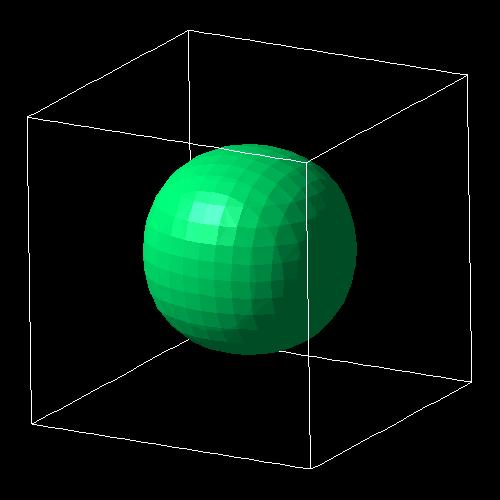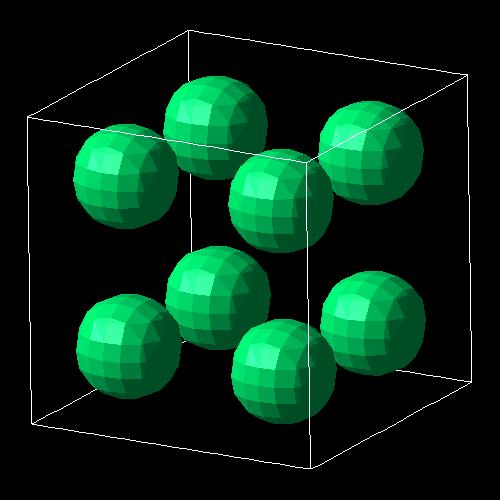### Intraband (=intersublevel) transitions

``` \$output-1-band-schroedinger   ...   intraband-matrix-elements = p     ! ```calculate intersublevel dipole moment < psif* | pz  | psii > and oscillator strength ffi```  ```

In this cubic QD with infinite barriers, optical intersublevel transitions are only allowed between states with odd difference quantum numbers along the same axes:
E111` <==> `E112/E121/E211```       1  <==>  2 /  3 /  4```
E111` <==> `E114/E141/E411```       1  <==> 21 / 22 / 23 ```E211` <==> `E311```               2  <==>  8```
E121` <==> `E131```               3  <==>  9```
E112` <==> `E113```               4  <==> 10 ...```

The following transitions are forbidden:
E111` <==> `E113/E131/E311```       1  <==>   8 /  9 / 10```
E211` <==> `E112/E121```           2  <==>   3 / 4```
E121` <==> `E211/E112```           3  <==>   2 / 4```
E112` <==> `E211/E121```           4  <==>   2 / 3```
`...`

## Energy levels in an idealistic 3D cubodial shaped quantum dot with Lx = Ly /= Lz

```-> 3DcuboidQD.in - ```10 nm x 10 nm x   5 nm QD

This time we use a similiar quantum dot as above but the lengths are Lx = Ly = 10 nm and Lz = 5 nm.

Therefore, the degeneracies of the eigenenergies are different. We expect the following:

En1,n2,n3 = hbar2 pi2 / 2me               ( n12 / Lx2 + n22 / Ly2n32 / Lz2 ) =
= 1.4462697 * 10-17 eVm2 ( n12 / Lx2 + n22 / Ly2n32 / Lz2 ) =
= 0.1446269  eV               ( n12           + n22 )  +  0.5785079 eV n32         )           (if Lx = Ly = 10 nm and Lz = 5 nm)

Generally, the energy levels are not degenerate, i.e. all energies are different.
However, some energy levels with different quantum numbers coincide, if the lengths along two or three directions are identical or
if their ratios are integers. In our cubic QD case, all three lengths are identical.
Consequently, we expect the following degeneracies:

• E111 = 0.86776 eV (ground state)
• E121 = E211 = 1.301642 eV
• E221 = 1.73552 eV = 2 E111    (This is a coincidence because Lx,y / Lz are integers and have the value 2.)
• E131 = E311 = 2.02478 eV
• E231 = E321 = 2.45866 eV
• E112 = 2.60329 eV = 2 E121    (This is a coincidence because Lx,y / Lz are integers and have the value 2.)
• E122 = E212 =
E141 = E411 = 3.03717 eV       (This is a coincidence because Lx,y / Lz are integers and have the value 2.)
• E331 = 3.18180 eV
• E222 = 2 E221 =                      (This is a coincidence because Lx,y / Lz are integers and have the value 2.)
E241 = E421    = 3.47105 eV    (This is a coincidence because Lx,y / Lz are integers and have the value 2.)
• E132 = E312 = 3.76030 eV
• E341 = E431 =
E232 = E322 = 4.19418 eV      (This is a coincidence because Lx,y / Lz are integers and have the value 2.)
• E151 = E511 = 4.33881 eV
• E142 = E412 =
E251 = E521 = 4.77269 eV      (This is a coincidence because Lx,y / Lz are integers and have the value 2.)
• E332 = 4.91731 eV
• E441 =
E242 = E422 = 5.20657 eV      (This is a coincidence because Lx,y / Lz are integers and have the value 2.)
• E113 = 5.49582 eV
• E123 = E213 = 5.92971 eV

nextnano³ numerical results for a 10 nm cubic quantum dot with
` - `0.50 nm grid spacing (left   column) and
` - `0.25 nm grid spacing (right column):
(The grid spacing is rather coarse (for 0.50 nm) but has the advantage that the calculation takes only a minute.)

Output file name:``` Schroedinger_1band/ev3D_cb001_qc001_sg001_deg001_dir_Kx001_Ky001_Kz001.dat```

``` num_ev: eigenvalue [eV]:         (0.50 nm grid)       (0.25 nm grid)   1      0.862425             0.866424      ```= E111```   2      1.291860             1.299191      ```(two-fold degenerate) = E121/E211```   3      1.291860             1.299191      ```(two-fold degenerate) = E121/E211```   4      1.721296             1.731958      ```= E221```   5      1.995828             2.017504      ```(two-fold degenerate) = E131/E311```   6      1.995828             2.017504      ```(two-fold degenerate) = E131/E311```   7      2.425263             2.450270      ```(two-fold degenerate) = E231/E321```   8      2.425263             2.450270      ```(two-fold degenerate) = E231/E321```   9      2.527557             2.584167      ```= E112```  10      2.956993             3.016933      ```(four-fold degenerate) = E122/E212/E141/E411```  11      2.956993             3.016933      ```(four-fold degenerate) = E122/E212/E141/E411```  12      2.956993             3.016933      ```(four-fold degenerate) = E122/E212/E141/E411```  13      2.956993             3.016933      ```(four-fold degenerate) = E122/E212/E141/E411```  14      3.129231             3.168583      ```= E331```  15      3.386428             3.449700      ```(three-fold degenerate) = E222/E241/E421```  16      3.386428             3.449700      ```(three-fold degenerate) = E222/E241/E421```  17      3.386428             3.449700      ```(three-fold degenerate) = E222/E241/E421```  18      3.660960             3.735246      ``` (two-fold degenerate) = E132/E312```  19      3.660960             3.735246      ``` (two-fold degenerate) = E132/E312```  20      4.090396             4.168013      ``` (four-fold degenerate) = E341/E431/E232/E322```  21      4.090396             4.168013      ``` (four-fold degenerate) = E341/E431/E232/E322```  22      4.090396             4.168013      ``` (four-fold degenerate) = E341/E431/E232/E322```  23      4.090396             4.168013      ``` (four-fold degenerate) = E341/E431/E232/E322```  24      4.151688             4.291319      ``` (two-fold degenerate) = E151/E511```  25      4.151688             4.291319      ``` (two-fold degenerate) = E151/E511```  26      4.581124             4.724086      ```(four-fold degenerate in theory) = E142/E412/E251/E521```  27      4.581124             4.724086      ```(four-fold degenerate in theory) = E142/E412/E251/E521```  28      4.622125             4.734676      ```(four-fold degenerate in theory) = E142/E412/E251/E521```  29      4.622125             4.734676      ```(four-fold degenerate in theory) = E142/E412/E251/E521```  30      4.794363             4.886326      ```= E332```  ...  34      5.121061             5.400036      ```= E441```  ...```

The following figures show the isosurfaces of the electron wave function (psi²) of a 10 nm x 10 nm x 5 nm cuboidal shaped quantum dot with infinite barriers for
` - `the ground state E111
` - `the   4th eigenstate E221```  - ```the   9th eigenstate E112
` - `the 14th eigenstate E331.
All these states are nondegenerate.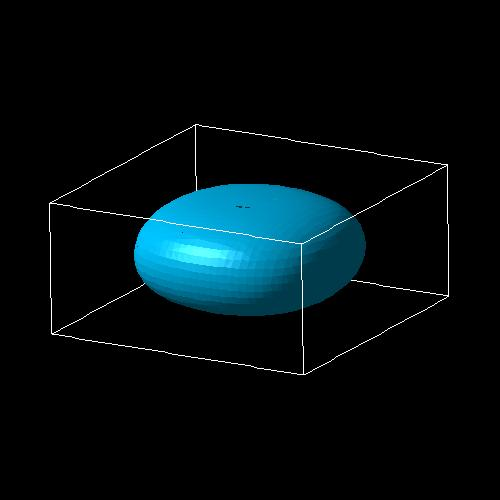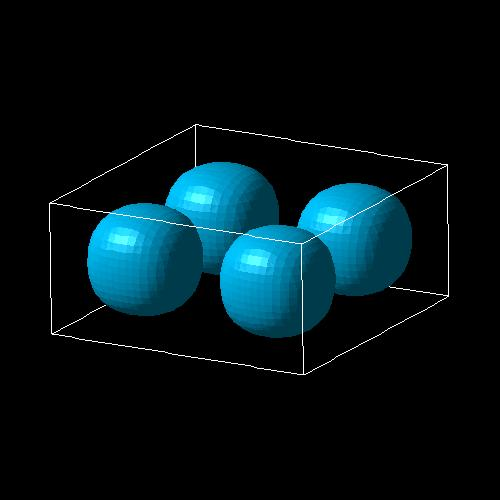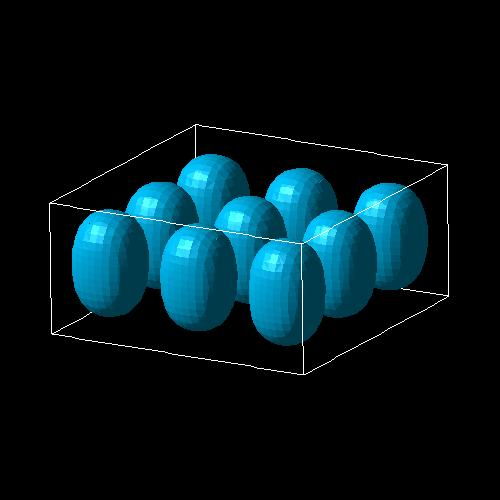• Please help us to improve our tutorial. Send comments to``` support [at] nextnano.com```.